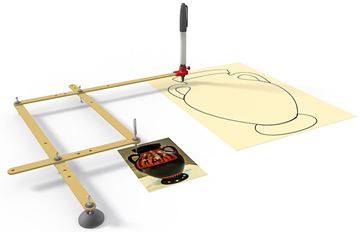Definition of

# ParallelogramA flat shape with 4 straight sides where opposite sides are parallel.

Also:
• opposite sides are equal in length, and
• opposite angles are equal (angles "A" are the same, and angles "B" are the same)

NOTE: Squares, Rectangles and Rhombuses are all Parallelograms!

Try moving any point below: